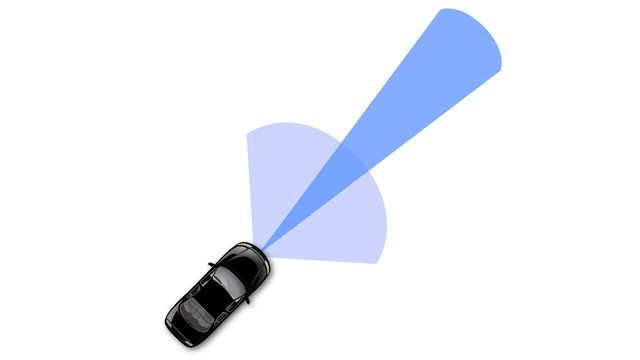# Delphi Develops Radar Sensor Alignment Algorithm for Automotive Active Safety System

## Challenge

Deliver a production automotive radar sensor alignment algorithm in four weeks

## Solution

Use MATLAB to develop the algorithm and use MATLAB Coder to generate production C code

## Results

• Generated C code as efficient as handwritten C code for this algorithm
• Development time halved
• Algorithm changes easily verified and coded in seconds

“MATLAB is my preferred tool because it speeds algorithm design and improvement. I can do the data analysis, algorithm development, algorithm visualization, and simulation in one place and then generate C code that is reliable, efficient, and easy for software engineers to integrate within a larger system.”

Liang Ma, DelphiDelphi’s multimode electronically scanning radar (ESR), which uses a single radar to provide wide coverage at midrange and high-resolution coverage at long range. Image courtesy of Delphi.

Automotive radar is an enabling technology for collision mitigation, blind-spot alerts, adaptive cruise control, and many other active safety features. Radar systems provide range, range rate, and azimuth information data on vehicles and other objects. The accuracy of this data depends on precise alignment of the radar sensor.

Delphi used MATLAB® and MATLAB Coder™ to accelerate the design, simulation, and implementation of a production radar sensor alignment algorithm.

“With MATLAB we can analyze data and design algorithms in one environment, so we can rapidly try out new ideas and then evaluate them with plots and statistical analysis,” says Liang Ma, Delphi system engineer. “Once we’ve verified an algorithm, we use MATLAB Coder to generate production C code that is as efficient as handwritten code.”

## Challenge

The radar sensor alignment algorithm executes more than 40 times every second while the vehicle is running. In 1 millisecond it must calculate the misalignment angle based on data provided by the radar sensor, as well as the vehicle speed, the sensor’s position on the vehicle, and its pointing angle.

In the past, Delphi system engineers handed off a MATLAB prototype algorithm to software engineers for implementation in C. This approach had several drawbacks. When the software engineers had a heavy workload, they often could not begin working on a C implementation for weeks. Communication was challenging because system engineers and offshore software engineers were separated by several time zones. Software engineers sometimes misinterpreted the prototype algorithm and delivered C code that failed to meet the design and performance requirements.

With active safety systems in high demand, Delphi had just four weeks to improve a radar sensor alignment algorithm for new radar products. They needed an approach that would enable system engineers to deliver their own production C code.

## Solution

Delphi adopted MATLAB and MATLAB Coder to develop and implement the radar sensor alignment algorithm.

Liang used MATLAB to analyze recorded sensor data captured from road testing a real vehicle. With huge amounts of testing data and the help of powerful MATLAB built-in functions, Liang realized and verified a radar sensor alignment algorithm that calculates sensor misalignment angles from raw radar detection and host vehicle speed. The algorithm computes the least squares solution to a system of linear equations. It also estimates the computed angle’s accuracy based on the residual of the least squares solution.

To verify the algorithm, Liang ran simulations using recorded sensor and vehicle data in MATLAB. He then used MATLAB scripts to process huge amounts of vehicle data to verify the accuracy of the sensor misalignment angle calculated by the algorithm.

He generated C code from the algorithm using MATLAB Coder. He verified the C code by calling a MEX function within the MATLAB testing code and comparing the results of the generated code with the results of the original MATLAB algorithm, completing each iteration in minutes.

Initially, the generated C code running on an ARM10 processor computed the misalignment angle in more than 3 milliseconds. Liang removed redundant logic, combined for-loops, and performed other optimizations in the MATLAB code until the generated code completed its computations in less than 1 millisecond, which met the throughput requirements.

On schedule, Liang delivered the verified C code for the improved algorithm to the software integration team for integration into the production system.

Delphi already uses this radar sensor alignment algorithm in active safety systems in production vehicles for several OEMs, with no reported defects.

Liang and his co-workers have used MATLAB and MATLAB Coder to design and implement several other production algorithms, including a target selection algorithm that uses fusion tracks information, camera vision objects, and host vehicle information to select appropriate targets for OEMs’ active safety features.

## Results

• Generated C code as efficient as handwritten C code for this algorithm. “The C code we generated with MATLAB Coder runs as fast as an earlier implementation of the algorithm that was hand-coded,” says Liang. “The generated code is also easy to integrate and free of defects—we never modify it.”

• Development time halved. “I developed the algorithm in three weeks, leaving just one week to implement it in C and verify it,” says Liang. “MATLAB Coder enabled me to complete the project on schedule. Hand-coding by a software engineer would have required up to four more weeks.”

• Algorithm changes easily verified and coded in seconds. “With our traditional approach, it could take a week for a software engineer to implement a change I had made to an algorithm,” says Liang. “With MATLAB and MATLAB Coder I can generate production C code myself in less than a minute, enabling me to evaluate new ideas quickly.”